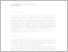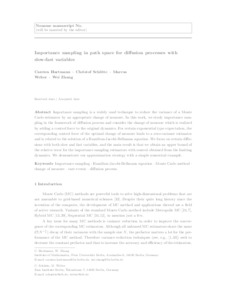Repository: Freie Universität Berlin, Math Department

# Importance sampling in path space for diffusion processes with slow-fast variables

Hartmann, C. and Schütte, Ch. and Weber, M. and Zhang, W. (2018) Importance sampling in path space for diffusion processes with slow-fast variables. Probab. Theory Rel. Fields, 170 (1-2). pp. 177-228. ISSN 0178-8051 (print) 1432-2064 (online)Preview
PDF - Accepted Version
366kB

Official URL: https://dx.doi.org/10.1007/s00440-017-0755-3

## Abstract

Importance sampling is a widely used technique to reduce the variance of a Monte Carlo estimator by an appropriate change of measure. In this work, we study importance sampling in the framework of diffusion process and consider the change of measure which is realized by adding a control force to the original dynamics. For certain exponential type expectation, the corresponding control force of the optimal change of measure leads to a zero-variance estimator and is related to the solution of a Hamilton-Jacobi-Bellmann equation. We focus on certain diffusions with both slow and fast variables, and the main result is that we obtain an upper bound of the relative error for the importance sampling estimators with control obtained from the limiting dynamics. We demonstrate our approximation strategy with a simple numerical example.

Item Type: Article SFB 1114 Preprint 02/2015 in arXiv:1502.07899 Mathematical and Computer Sciences > Mathematics > Mathematical Methods Department of Mathematics and Computer Science > Institute of MathematicsDepartment of Mathematics and Computer Science > Institute of Mathematics > BioComputing GroupDepartment of Mathematics and Computer Science > Institute of Mathematics > Cellular Mechanics GroupOther Institutes > Matheon 1321 Carsten Hartmann 13 Sep 2013 18:58 17 Jan 2020 10:46

Repository Staff Only: item control page